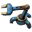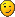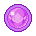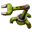## Modulo @ Arithmetic Combinator

Anything related to the content on our wiki (https://wiki.factorio.com/)

Moderator: Bilka

burninghey
Fast InserterPosts: 105
Joined: Fri Sep 14, 2018 2:06 am
Contact:

### Modulo @ Arithmetic Combinator

I'm not a math expert, but I think i have discovered an error @ https://wiki.factorio.com/Arithmetic_combinator
Could fix it myself of course, but I do not want to enter wrong informationThis one:
Modulo, indicated using % as it is in most programming languages, is the remainder after division. For example, 13 % 3 is 1 (13 = 4 * 3 + 1). This can be used, for example, to separate out individual digits of a number for use in building visual indicators, along with division:
- While the example "13 % 3 is 1 (13 = 4 * 3 + 1)" is easy, the following confuses me:
(24321 / 10000) % 10 = 2
(24321 / 1000) % 10 = 4
(24321 / 100) % 10 = 3
(24321 / 10) % 10 = 2
(24321 / 1) % 10 = 1
just seems to be wrong. There is an older version of that part:
https://wiki.factorio.com/index.php?tit ... did=138178
Modulo: marked somewhat confusingly as %, is the remainder after division. For example 10 modulo 3 is 1 (3*3=9, remainder 1). This can be used for example to separate out thousands, hundreds, tens and ones for use in building visual indicators. e.g.

24321 modulo 10000 = 4321
24321 modulo 1000 = 321
24321 modulo 100 = 21
24321 modulo 10 = 1
which seems to be correct and nowhere the same as above.

boskid
Factorio StaffPosts: 1529
Joined: Thu Dec 14, 2017 6:56 pm
Contact:

### Re: Modulo @ Arithmetic Combinator

Both current and old are correct.

valneq
Smart InserterPosts: 1100
Joined: Fri Jul 12, 2019 7:43 am
Contact:

### Re: Modulo @ Arithmetic Combinator

burninghey wrote:
Sun Nov 08, 2020 2:33 am
- While the example "13 % 3 is 1 (13 = 4 * 3 + 1)" is easy, the following confuses me:

(24321 / 10000) % 10 = 2
(24321 / 1000) % 10 = 4
(24321 / 100) % 10 = 3
(24321 / 10) % 10 = 2
(24321 / 1) % 10 = 1
This is correct if you interpret the division symbol as pure integer division, i.e. rounding down and thus resulting in an integer. A more explicit way of writing this would be
floor(24321 / 10000) % 10 = 2
floor(24321 / 1000) % 10 = 4
floor(24321 / 100) % 10 = 3
floor(24321 / 10) % 10 = 2
floor(24321 / 1) % 10 = 1
Otherwise, you have to be more explicit in how you define the modulo operation on floating point numbers.

Pi-C
Smart InserterPosts: 1086
Joined: Sun Oct 14, 2018 8:13 am
Contact:

### Re: Modulo @ Arithmetic Combinator

valneq wrote:
Sun Nov 08, 2020 4:52 am
This is correct if you interpret the division symbol as pure integer division, i.e. rounding down and thus resulting in an integer.
Interesting. I've looked at a mod yesterday that had code like this:

Code: Select all

``````local runs = math.max(1,(global.firecount or 0)/5) + (global.fractional_tick or 0)
global.fractional_tick = runs % 1
``````
This didn't make sense (in my opinion x%1 would always be 0). So I tested it on my calculator -- which failed because it expects integers for modulo. However, when I executed this directly in Lua from the command line, it did return the fractional part (i.e. 0<x<1). Guess I'll have to check how Factorio handles this when I'm back at the computer where it's installed. Whatever the outcome, it would be a good idea to explicate whether Factorio's Lua implementation will use floating point values with modulo directly or whether it will silently convert them to integers.
A good mod deserves a good changelog. Here's a tutorial (WIP) about Factorio's way too strict changelog syntax!

valneq
Smart InserterPosts: 1100
Joined: Fri Jul 12, 2019 7:43 am
Contact:

### Re: Modulo @ Arithmetic Combinator

Well, considering that mods are written in Lua, and Lua defines modulo like this (source):
Lua 5.1 Reference Manual wrote: a % b == a - math.floor(a/b)*b
you get exactly what you observed.

SoShootMe
Fast InserterPosts: 161
Joined: Mon Aug 03, 2020 4:16 pm
Contact:

### Re: Modulo @ Arithmetic Combinator

burninghey wrote:
Sun Nov 08, 2020 2:33 am
I'm not a math expert, but I think i have discovered an error @ https://wiki.factorio.com/Arithmetic_combinator
Could fix it myself of course, but I do not want to enter wrong informationThis one:
Modulo, indicated using % as it is in most programming languages, is the remainder after division. For example, 13 % 3 is 1 (13 = 4 * 3 + 1). This can be used, for example, to separate out individual digits of a number for use in building visual indicators, along with division:
- While the example "13 % 3 is 1 (13 = 4 * 3 + 1)" is easy, the following confuses me:
(24321 / 10000) % 10 = 2
(24321 / 1000) % 10 = 4
(24321 / 100) % 10 = 3
(24321 / 10) % 10 = 2
(24321 / 1) % 10 = 1
just seems to be wrong.
The preceding section explains that division is truncated; perhaps more explicitly linking the two, up front, would help make the example clearer? Something like:

"This can, for example, be combined with truncated division as described above to separate out individual digits ..."

Bilka
Factorio StaffPosts: 2785
Joined: Sat Aug 13, 2016 9:20 am
Contact:

### Re: Modulo @ Arithmetic Combinator

The division is truncated, as explained in this thread and on the page. I added the extra note suggested by SoShootMe (thank you!) to make this more clear on the page.

Note that the page is only about the circuit network. The Lua modulo operator that was mentioned in this thread behaves differently. Lua modulo is documented in the Lua documentation, as mentioned by valneq (direct link: https://www.lua.org/manual/5.2/manual.html#3.4.1).
I'm an admin over at https://wiki.factorio.com. Feel free to contact me if there's anything wrong (or right) with it.

burninghey
Fast InserterPosts: 105
Joined: Fri Sep 14, 2018 2:06 am
Contact:

thank you!